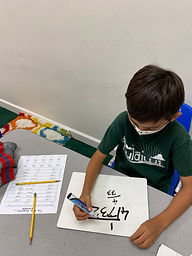## Ms. Kylie

### Target 1​

###### Lesson Type:

Continuation

Measurment

:

Relationships

Understand the relationship that exists between area and perimeter.

###### 1:

Understand that figures with the same area can have different perimeters (and vice versa).

###### 2:

Identify patterns and strategies involved in working with area and perimeter.

###### 3:

Explain the strategy for making the smallest perimeter.

###### 4:

Explain the strategy for making the largest perimeter.

4th

###### Vocabulary:

Area, Perimeter, Length, Width

Activities:

• Students were given the measurements of an irregular shape and found the perimeter and the area. Then they created a different shape with same area but different perimeter.

• Students completed an activity to draw different size workshops for santa's elves. To draw each elf's workshop they were given information about the area and perimeter and used that information to draw the workshop.### Home Exploration

###### Challenge Problem:

The area of a shape is 48 squared feet and the perimeter is 28 feet. What is the meausrement of each side of the shape?

###### Guiding Questions:

-If area of a shape is L x W, then what are the factors of 48?

-If perimeter is the meaursement of all sides, then what factors of 48 when each factor is halved and then added all together will equal 28 ft?

9 ft. by 6 ft.## Absent Students:

### Target 2

:

###### 1:

Understand the role of place-value in division strategies.

###### 2:

Use the standard algorithm for solving multi-digit division equations.

###### 3:

Understand that there can be a remainder in a division problem.

4th

###### Vocabulary:

Dividend, Divisor, Remainder

Activities:

• Students learned to solve long division problems using the standard algorithm.

• Students understood when dividing using the standard alogrithm they are seeing how many times the divisor can be divided by each digit in the dividend.### Home Exploration

###### Guiding Questions:### Target 3

:

###### Vocabulary:

Activities:### Home Exploration